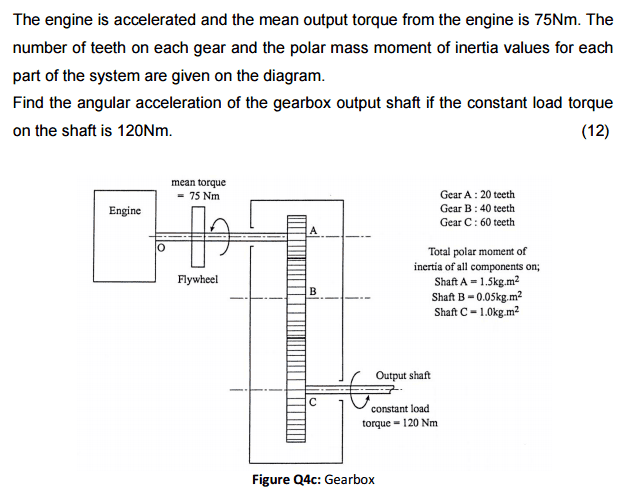# Drive line inertia and torsional effects exam question

• Alistair McCheyne

#### Alistair McCheyneHi can anyone help me with this question on inertial forces in gears?
Cheers
Ali :)

#### Attachments

For every shaft $i$, you get:
$$\sum T_i = I_i \alpha_i$$
And for every gear $i$ connected to gear $j$, you get:
$$\frac{T_{i\ out}}{T_{j\ in}} = \frac{N_i}{N_j}$$
$$\frac{\alpha_i}{\alpha_j} = \frac{N_j}{N_i}$$

This gives you 7 equations with 7 unknowns ($T_{A\ out}$, $T_{B\ in}$, $T_{B\ out}$, $T_{C\ in}$, $\alpha_A$, $\alpha_B$, $\alpha_C$).

i would be tempted to apportion an effective moment of inertia to shafts B & C (this takes into account the relative rotation rates)

Select an arbitrary rotation rate for shaft A (say 10 rad/sec)
Calculate the rotational KE of each shaft at this rate
The effective moment of inertia of shafts B and C you calculate by comparing the KE values to that of shaft A
For example :
The KE of shaft A at 10 rad / sec = 75 joules
The KE of shaft B at 5 rad / sec = 0.625 joules, so its effective moment of inertia = 1.5 * ( 0.625 / 75 ) = 0.0125 kg . m^2
Repeat for shaft C then add A, B and C for total effective moment of inertia of the system